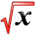# Separable EquationsThis article is part of the MathHelp Tutoring Wiki

Definition: A separable equation is a first order equation that can be factored as a function of x times a function of y. dy/dx=g(x)*f(y)

General Solution: Q: If we know dy/dx=g(x)*f(y), we want to write y in terms of x.

Solution strategy:

Assume f(y) ++=++ 0 and h(y)=1/f(y)

so dy/dx=g(x)*f(y)=g(x)/h(y)

Formally cross multiplication

h(y)*dy=g(x)*dx

Integrate ∫ h(y)dy=∫ g(x)dx

Q1: dy/dx=x2*y, then what is y in terms of x?

A: if y ++=++ 0,

dy/y=x2*dx

∫ dy/y=∫ x2*dx

ln|y|=x3/3+c

y=__+__ex3/3+c

Q2:

dy/dx=(6x2)/(2y+cosy)

A:

∫ (2y+cos(y))dy=∫ 6x2*dx

y2+sin(y)=2x3+c